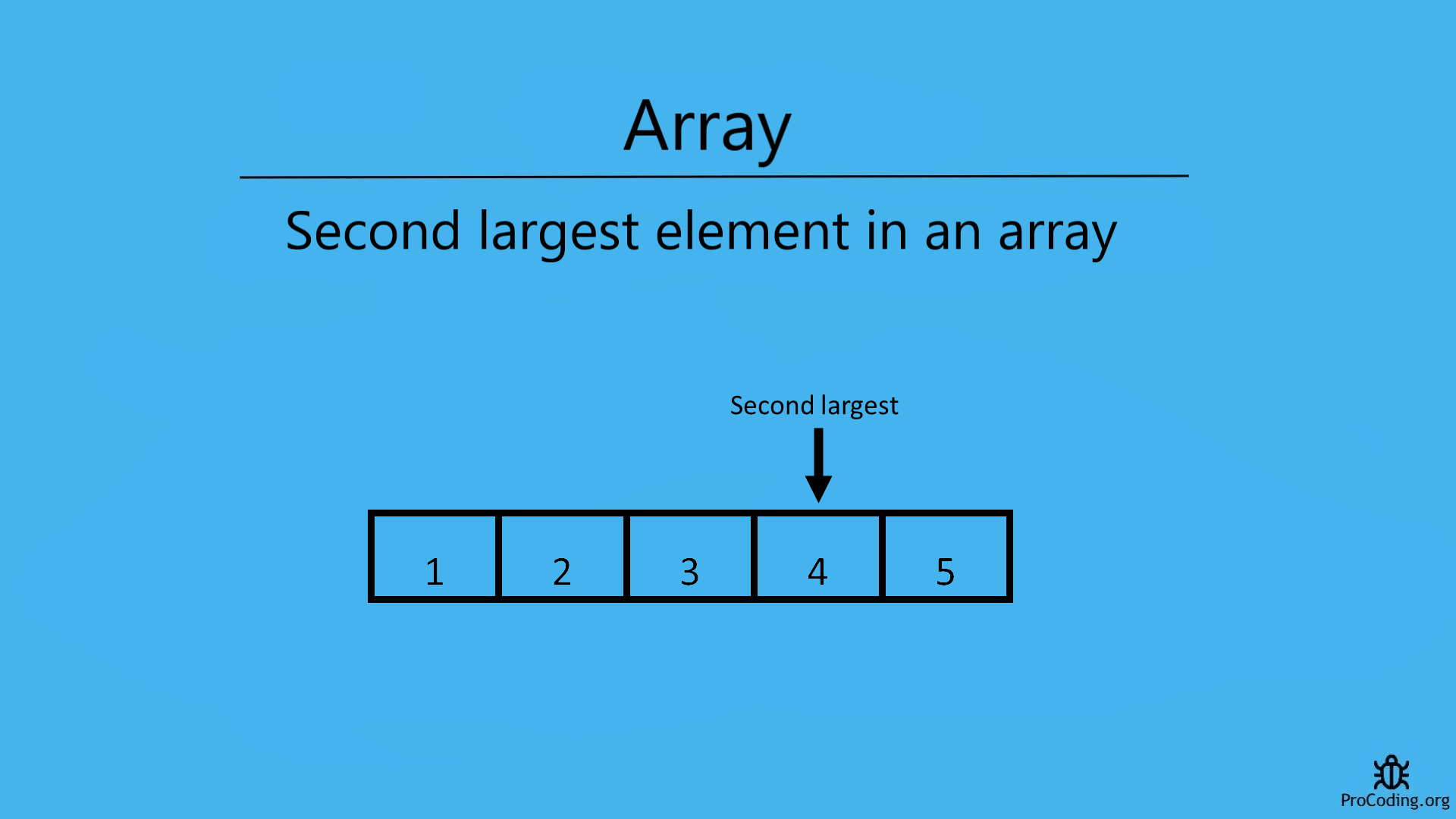# Second largest element in an array

Shriya Madan Category: Data StructuresTags: datastructures array

Given an array write a program to find the second largest element in an array by using the efficient possible solution.
Learn the logic to find the second largest number efficiently in an array using a single traversal.So in this post, we are going to learn the logic behind finding the second largest element in an array.

##### Example -
```Input: [4, 2, 1, 3, 5]
Output: 4

Input: [200, 200, -50, 10]
Output: 10```

### Approach 1

We will first discuss an easy way to solve this problem-

``````1. Sort the given array.
2. Compare elements in the array starting from the last element, i.e. index
n-1, till you find an element that is lesser than the largest element.
This will test for the cases when the array has repeated elements.
3. Print the second largest element.``````
``````#include <bits/stdc++.h>
using namespace std;

int secondLargest(int arr[], int n) {
int i;
sort(arr, arr + n);

for (i = n - 2; i >= 0; i--) {
if (arr[i] != arr[n - 1]) {
return arr[i];
}
}

}

int main() {
int n = 5;
int arr[] = {4, 2, 1, 3, 5};

cout << "The second largest element is: " << secondLargest(arr, n);
return 0;
}``````
``````def second_largest(arr):
arr.sort()
for i in range(len(arr)-2, 0, -1):
if arr[i] != arr[i - 1]:
return arr[i]

arr = [4, 2, 1, 3, 5]
print("The second largest element is: {0}".format((second_largest(arr))))``````
``````function secondLargest(arr) {
arr.sort();
for (let i = arr.length - 2; i >= 0; i--) {
if (arr[i] != arr[i - 1]) {
return arr[i];
}
}
}

arr = [4, 2, 1, 3, 5];
console.log(`The second largest element is: \${secondLargest(arr)}`);``````

#### Output

Output

`The second largest element is: 4`

The time complexity of sorting is high and hence this approach is not suitable for an array containing a large number of elements

### Approach 2:

This approach is better as it will do only a single traversal of the array. To keep track of the second largest element, we will be identifying the first largest element. Hence no sorting needed, its time complexity will be less. Following are the steps to solve this problem:

``````1. To keep track of the first largest element, we need to initialize two variables- max and second-max.
2. Run a loop to traverse the array with two conditions:
i) If the current element in the array, arr[i], is greater than max.
Then update max and second-max as,
second-max = max
max = arr[i]
ii) If the current element is in between max and second-max,
then update second-max to store the value of the current variable as
second-max = arr[i]
3. Print the value of second-max``````
``````#include<iostream>
using namespace std;

int secondLargest(int arr[], int n) {
int max, i, secondMax;
max = arr;

for (int i = 0; i < 5; i++) {
if (arr[i] > max) {
secondMax = max;
max = arr[i];
} else if (arr[i] > secondMax && arr[i] < max)
secondMax = arr[i];
}
return secondMax;
}

int main() {
int n = 5;
int arr[] = {4, 2, 1, 3, 5};

cout << "The second largest element is: " << secondLargest(arr, n);
return 0;
}``````
``````def second_largest(arr):
max_num = arr
second_max = float('-inf')
for i in range(len(arr)):
if arr[i] > max_num:
second_max = max_num
max_num = arr[i]
elif arr[i] > second_max and arr[i] < max_num:
second_max = arr[i]
return second_max

arr = [4, 2, 1, 3, 5]
print("The second largest element is: {0}".format((second_largest(arr))))``````
``````function secondLargest(arr) {
let maxNum = arr;
secondMax = Number.NEGATIVE_INFINITY;
for (let i = 0; i < arr.length; i++) {
if (arr[i] > maxNum) {
secondMax = maxNum;
maxNum = arr[i];
} else if (arr[i] > secondMax && arr[i] < maxNum) {
secondMax = arr[i];
}
}
return secondMax;
}

arr = [4, 2, 1, 3, 5];
console.log(`The second largest element is: \${secondLargest(arr)}`);``````

#### Output

Output

`The second largest element is: 4`

AUTHOR